# Excel 2010: Apply Basic Formulas And Conditional Formatting

Formulas are the heart of Excel. With huge list of built-in formula and functions, Excel stands out from other datasheet handling applications. Formulas are used for calculating/analyzing data based on values in designated cells. It supports trigonometric, statistical and other functions. You can also create a new rule, or constraint to apply over your datasheet. This post covers writing formulas and applying conditional formatting on a basic level.

For Instance: We will start off with creating simple worksheet of students, which includes; Name of the student, and obtained Marks in their respective courses.We need to add two new columns which shows which grade the student has secured and status of the student which shows whether he is pass or fail. So we will add two new columns having names Grade and Status respectively.We will write a formula which evaluates the grade student has secured. By considering if secured marks are greater than/equal to 80, then he falls in A grade, greater than or equal to 75 secured B+ grade and so on and student who obtained less than 60 marks secured D grade.

The basic syntax of the formula is this:

=IF(Criteria, Action if Criteria Met, Other Action)

At the first row of Grade column, formula goes like this.

=IF(D2>=80,”A”, IF(D2>=75, “B”, IF(D2>=70, “B+”, IF(D2>=70,”B”, IF(D2>=65,”C+”, IF(D2>=60, “C”,”D”))))))

In the line of code, we are writing formula for D2 position which actually contains 60 marks in Grade column. From the formula (=IF D2 >=80,”A”,…..) inferring that if D2 position contains value which is greater than or equal to 80. the Grade ‘A’ would be printed in the designated cell and if marks are greater than or equal to 75, ‘B’ would show and so on. When we put in this formula, it will show the grade student has secured according to the formula constraints. When you will drag down the cross symbol to the end of the column, the formula will be applied to all respective cells.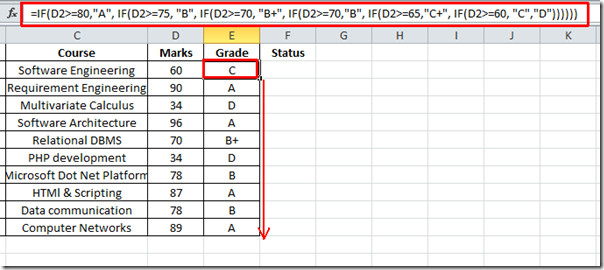Now at the first row of the Status column, we will write formula which goes like this:

=IF(E2=”A”,”Pass”,IF(E2=”B”,”Pass”,IF(E2=”B+”,”Pass”,IF(E2=”C”,”Pass”,IF(E2=”C+”,”Pass”,IF(E2=”D”,”Fail”))))))

From the above formula we can infer that if E2 position contains D, the word Fail will be seen in the Status column and in all other conditions Pass would show. Drag down the cross symbol to the end of the column to apply the formula over all the respective cells.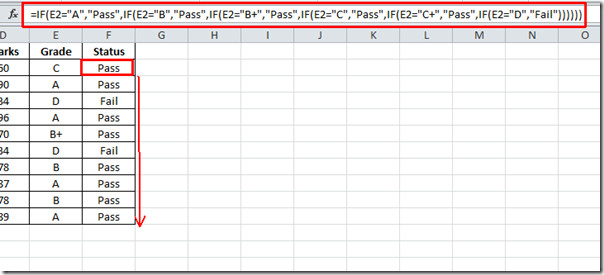As shown in the above screenshot, where the grade is ‘D’ the word ‘Fail’ can be seen in the corresponding cell.

Conditional Formatting allow users to show only specific data that meets a certain criteria or condition. Navigate to Home tab and click Conditional Formatting button, you will see list of different options.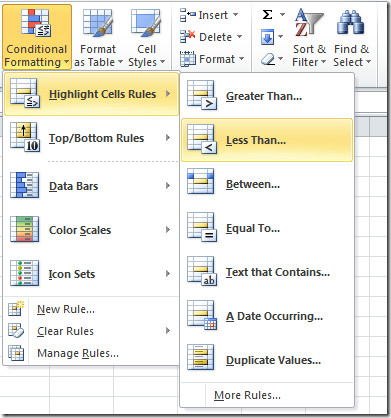Select the Marks column, go to conditional formatting and in Highlight Cells Rules, click Less than. Enter the value 60 and select any formatting style. On writing valid value, the selected column will automatically be updated with selected formatting style. Click OK to apply.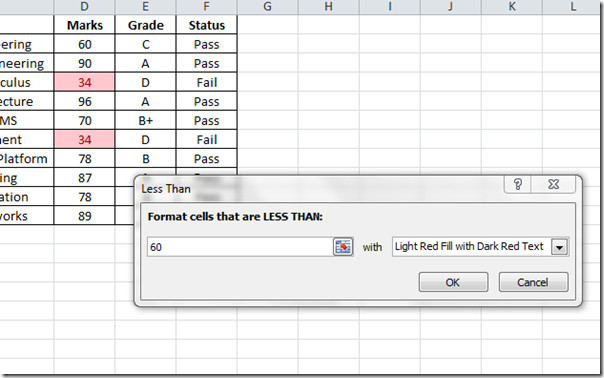Apply conditional formatting styles over all the data to make portion of datasheet more prominent and clear.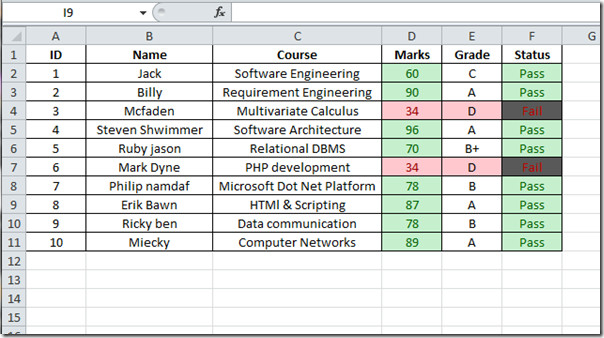1.Greg Quick says:

How Can I do this? If Column B is Highlighted Yellow place an “x” in column A.

2.Carey Ginn says:

I am having trouble with an excel formula. If(j24=X,”Y”,””) and it keeps giving me “N”. In a previous row I used IF(J21=x,”N”,””)

3.sahar says:

thank u for helping….wonderful explanation

4.Carol says:

•Rubin Gurabardhi says:

Hi there Carol,

In cell D7 input the following formula:

=IF(G7=3000643,IF(G7=3001283,”Lamb01″,0))

5.~Doc says:

No way, Jose?

6.david says:

IF(or(AND(AND(C3>=35,D3>=35,E3>=35,F3>=35,G3>=35,H3>=35),I3>=210,I3=35,D3>=35,E3>=35,F3>=35,G3>=35,H3>=35),I3>=301,i=35,D3>=35,E3>=35,F3>=35,G3>=35,H3>=35),I3>=360,I3<=600),"first","fail")) WWhat is error in this formula please rectify or suggestion

7.Ed says:

Conditional Formatting issue: I am using a formula that references a cell in another sheet in the same workbook (example: =B23other sheet!H4). When I apply it, it works fine and does what it is supposed to do. After saving and reopening the file the formatting is GONE. Cant I reference a cell from another sheet? If so, why is the conditional formatting disappearing? I tested it with a formula comparing cells on the same sheet – no issue after saving. The issue only appears when I reference the other sheet.

8.Ace says:

I wish to shade or format a cell (alignment, strikethrough) based on the value of another cell. The cell I need to format already contains a lookup value from another sheet.

9.Lutchy says:

I have a column that shows whether a person in a male or female and another column which displays the age. So I woul like to test whether a peson’ age >= 65 AND if this person is male or >= 60 AND female (reason females retire at age 60 and males retire at age 65) than the result if true should be Pensioner if fales Not Pensioner.

•Iulian says:

Hi,
for a table where the column heads (A1, B1, C1, D1) are:
NAME SEX AGE STATUS
in column under the STATUS (D2)

the formula would be

=IF(AND(B2=”male”;C2>=65)=TRUE;”Pensioner”;IF(AND(B2=”female”;C2>=605)=TRUE;”Pensioner”;”not pensioner”))

10.Sanisha says:

cell E6 turns into a C+ nmark with this formula ,, not a B+.

11.sam says:

what if i want to use if and and function how does it work ?? for example, based on Man, teenager and senior, if he’s a senior and he has more than \$1000 in his bank account, “rich” should be the outcome in the designated cell, and if he’s a teenager and he has an amount between \$200 and \$500 in his bank account “Moderate” should be the outcome….and so on like multiple IF AND formula!! how does that work

12.Steve Goode says:

I need to show different cell shading according to the figure in the cell. However, I want to show the same cell shading for a positive rating as for a negative rating e.g. greater than +50 might be shaded red and greater than -50 (less than really) should also be shaded red. I don’t seem to be able to do this with conditional formatting alone. Any thoughts?

13.Ricky Barnawal says:

hay
i need samall format for codinational formt for equil Serial Number in cell colour change
just like A1= 25125 F, A85 =25125 F after Colur Change

14.Iulian Trisca says:

Does anyone knows a way to format a cell depending of the outcome of an if function?
and i don’t mean conditional formatting. the issue i have is my IF&VLOOKUP function combination evaluates whether a cell in a source sheet is empty or not and then based on that it calculates a value or copies the value from the source sheet. As this is an automatic update i would like to show to the viewer if the value in the cell has been calculated or extracted from the source sheet by formatting it differently.

15.Anonymous says:

Did anyone else notice the error in the formula for “B” grades?  The way it’s written, the student with a 70 gets a “B+” and the student with 78 gets a “B”.  It should be changed to:
=IF(D2>=80,”A”, IF(D2>=75, “B+”, IF(D2>=70,”B”, IF(D2>=65,”C+”, IF(D2>=60, “C”,”D”)))))

•Truth says:

that is due to the order of the conditional settings. easily fixed

16.Rubin Gurabardhi says:

Hi there,

Could you help me on the following:

If i have two columns A and B. In the first A lets say that we have the currency for example EUR, USD, CHF, in the other column B I have the Values in Original Currency. I want to add another column C that gives me the Equivalent in EUR.
=IF(A2=”EUR”,b2*rate!\$B\$1,IF(A2=”USD”,-B2*rate!\$B\$2,IF(A2=”CHF”,-B2*rate!\$B\$3,-K2))). The sheet rate! has the the daily exchange currency ratio.

Best regards,

Rubin

17.HB says:

Thanks for this very helpful tip. I was looking for something to change colour of text for values below certain number.

18.Paul says:

There is a Quick Reference Card available for VLOOKUP here
http://officeimg.vo.msecnd.net/en-au/files/818/530/AF101984660.pdf
Although the purpose of the page is conditional formatting, it would benefit from using a less clumsy formula.
For something like student grades, it is important that your grade lookup is in order (ie: lowest grade to highest grade) and you set the “range lookup” field to true (or 1)

•Cbhatikar says:

Suppose you want same color for the full row after conditional formatting, what do i do???
Pls help…

19.Paul says:

For something like this, you really should be using VLOOKUP instead of IF
The example above is promoting bad practice

20.Lauren says:

Mine worked fine..

•Anand says:

great

21.Jose Corrales says:

Hey,
If you are going to post something like this on the web, at least try them out, I just tried the very same freaking formula on the same freaking format you are showing it and the motherfucking thing does not work!

•Just a guy says:

Grow up and learn how to express yourself like a man.

This site uses Akismet to reduce spam. Learn how your comment data is processed.